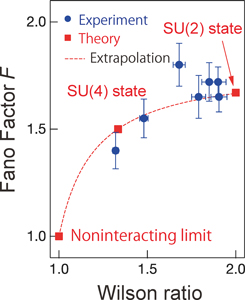# ISSP - The institute for Solid State PhysicsFont Size: (S) / (M) / (L)

- Activity Report 2017 -

Kato Group

Crossover between Kondo States in Carbon Nanotube Quantum Dot Observed by an Ultrasensitive Noise MeasurementFig. 1. Two aluminum electrodes (cyan) are attached to a carbon nanotube quantum dot (yellow). Electric current and its noise through them are investigated. Electrons confined in the carbon nanotube dot hold not only the spin degree freedom but also a degree of freedom due to the direction of electron motion (red arrows wrapped around the nanotube), which can bring a rich variety of the Kondo state.Fig. 2. Fano factor as a function of the Wilson ratio.

We have realized the SU(2) and SU(4) Kondo many-body states in a carbon nanotube quantum dot made by the cutting-edge nanofabrication technology, and finely controlled a crossover phenomenon between the two Kondo states by applying magnetic fields. Our very accurate current noise measurement successfully elucidated that the local moment structure within the quantum dot can be adjusted to increase the quantum fluctuation of the Kondo state .

The Kondo effect is a typical quantum many-body phenomenon, where electrons gather around the moment of a localized electron and form a new state (Kondo state) together and quantum fluctuation of the angular moment of the electrons plays the essential role in formation of the Kondo state. The electrons confined in the carbon nanotube quantum dot hold not only spin but also additional degrees of freedom, namely, the direction of electron motion (see Fig. 1), which brings a variety to the Kondo state. However, it is not easy to distinguish the SU(2) and SU(4) Kondo states by power laws emerging in observed current as function of temperatures or applied bias-voltages, because both of the Kondo states are governed by the local Fermi liquid. The key idea of our work is to observe and analyze current noise. The Fano factor given by the ratio of the nonlinear current noise and the nonlinear current represents a strength of the local-Fermi-liquid interaction, which indicates a strength of quantum fluctuation of the Kondo ground state. The Fano factor also shows an intrinsic value corresponding to the local moment which causes the Kondo state.

Figure 2 shows the observed Fano factor F as a function of the Wilson ratio R. In our experiment, the Wilson ratio is controlled by applied magnetic field. The values of the Wilson ratio corresponding to experimentally applied magnetic field are estimated by numerical renormalization calculation . The Wilson ratio are varied from R = 4/3 at the zero magnetic field, where the SU(4) Kondo state is realized, to R = 2 , the large magnetic field limit where the SU(2) Kondo state is realized. In Fig. 2, the Fano factor varies from F = 1.4 ± 0.1 at the zero field to F = 1.7 ± 0.1 at a large field. These observations well agree with F = 3/2 and 5/3 theoretically predicted for the SU(2) and SU(4) Kondo states, respectively [3, 4].

In this research, we have experimentally demonstrated that the strength of quantum fluctuation depends on the type of Kondo states. Both the Kondo effect and quantum fluctuation are central issues in physics. Our result brings a deeper understanding of quantum many-body phenomena, and opens a new way to control quantum fluctuation and to explore new function of materials.

References

•  M. Ferrier, T. Arakawa, T. Hata, R. Fujiwara, R. Delagrange, R. Deblock, Y. Teratani, R. Sakano, A. Oguri, and K. Kobayashi, Phys. Rev. Lett. 118, 196803 (2017).
•  Y. Teratani, R. Sakano, R. Fujiwara, T. Hata, T. Arakawa, M. Ferrier, K. Kobayashi, and A. Oguri, J. Phys. Soc. Jpn. 85, 094718 (2016).
•  R. Sakano, T. Fujii, and A. Oguri, Phys. Rev. B 83, 075440 (2011).
•  R. Sakano, A. Oguri, T. Kato, and S. Tarucha, Phys. Rev. B 83, 241301(R) (2011).

Authers

• R. Sakano, M. Ferriera, T. Arakawab, T. Hatac, K. Kobayashib, Y. Teratanid, and A. Ogurid
• aUniversité de Paris-Sud
• bOsaka University
• cTokyo Institute of Technology
• dOsaka City University